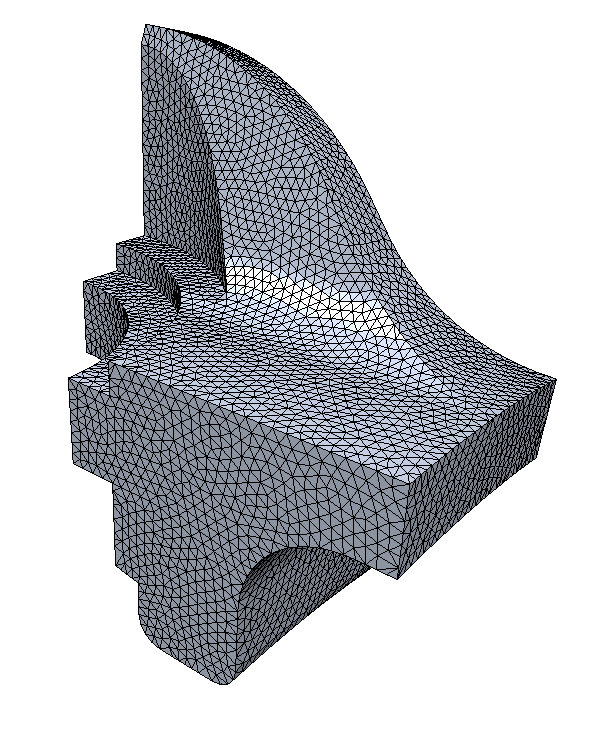Interactive HTML Slides
for Geometry Processing
using the PMP library
Graphics & Geometry Group
Bielefeld University

# How to use these HTML slides

• Use the cursor keys left/right to navigate through the slides
• Click page number (bottom right) to open navigation menu
• Press f/ESC to enter/leave fullscreen mode
• Press o or ESC to enter/leave overview mode
• Double-click an item (e.g. an image) to zoom in/out.

# Interactive Demo Applications

• The demos are written in C++ using the PMP library
• They are cross-compiled to Javascript using emscripten
• They require support for WebGL 2
• See https://caniuse.com/webgl2
• The demos work nicely on Chrome, Chromium, or Firefox
• They do not run on Apple Safari in MacOS and iOS

# Loop Subdivision

• Subdivision scheme for triangle meshes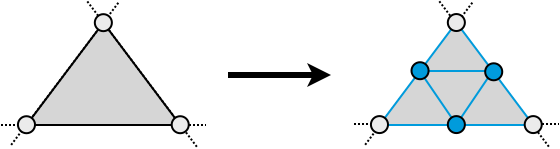• Generates $$C^2$$ continuous limit surfaces:
• $$C^1$$ for extraordinary vertices (valence ≠ 6)
• $$C^2$$ continuous everywhere else

# Loop Subdivision Rules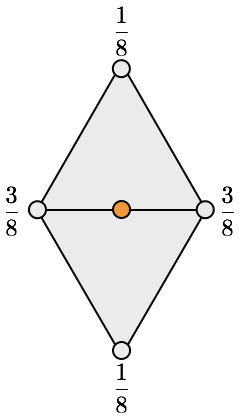New edge vertices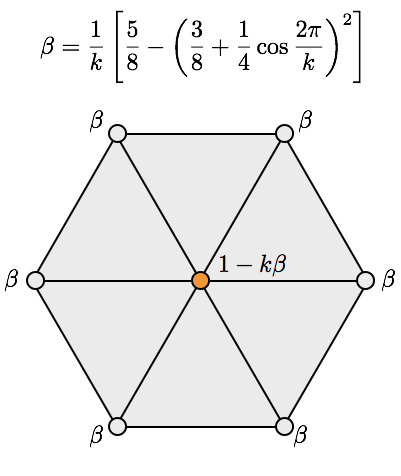Update old vertices (valence $$k$$)

# Generalized Catmull-Clark Subdivision

• Subdivision scheme for arbitrary polygons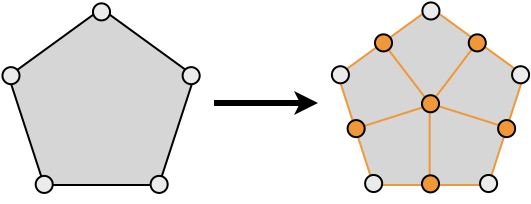• Connect new face points to edge-vertex-edge triple
• Turns all polygon faces into quads
• Generates $$C^2$$ continuous limit surfaces:
• $$C^1$$ for extraordinary vertices (valence ≠ 4)
• $$C^2$$ continuous everywhere else

# Catmull-Clark Rules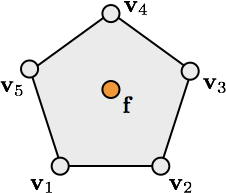New face vertices

$\vec{f} = \frac{1}{n} \sum_{i=1}^n \vec{v}_i$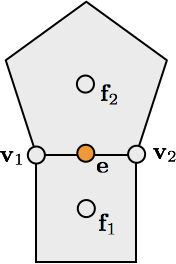New edge vertices

$\vec{e} = \frac{1}{4} \left(\vec{v}_1 + \vec{v}_2 + \vec{f}_1 + \vec{f}_2\right)$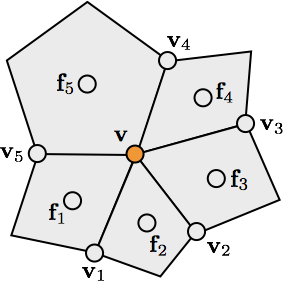Update old vertices (valence $$k$$)

$\vec{v} = \frac{k-2}{k} \vec{v} + \frac{1}{k^2} \sum_{i=1}^k \vec{v}_i + \frac{1}{k^2} \sum_{i=1}^k \vec{f}_i$

# Diffusion Flow on Meshes

• Continuous PDE: $$\frac{\partial \vec{x}}{\partial t} \;=\; \lambda \Delta \vec{x}$$
• Explicit integration per vertex: $$\vec{x}_i \leftarrow \vec{x}_i + \delta t \, \lambda \Delta \vec{x}_i$$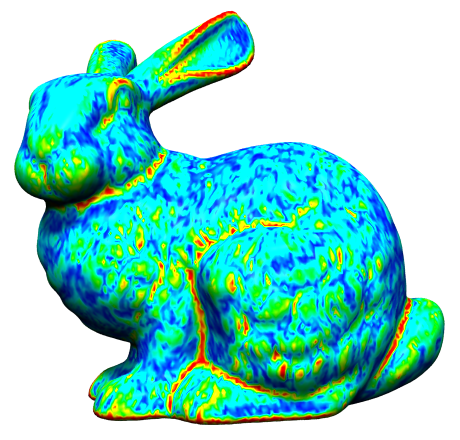0 iterations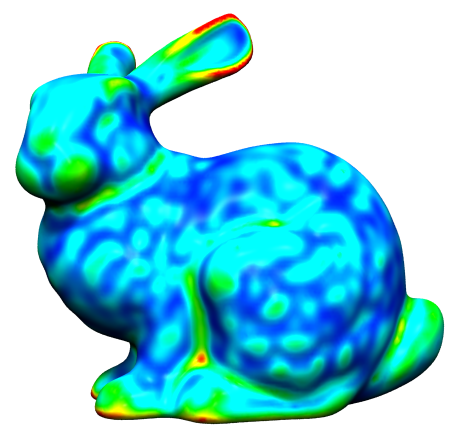10 iterations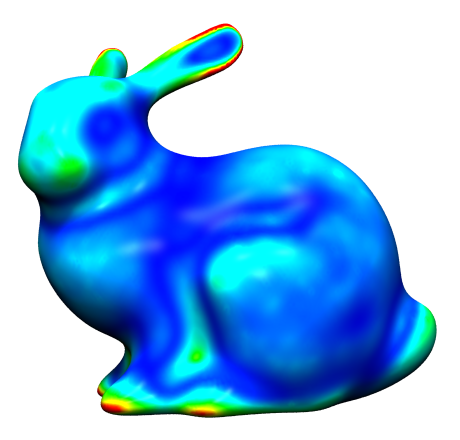100 iterations

# Uniform Laplace Discretization

$\laplace \vec{x}\of{v_i} \;:=\; \frac{1}{\abs{\set{N}_1\of{v_i}}} \sum_{v_j \in \set{N}_1\of{v_i}} \left( \vec{x}\of{v_j} - \vec{x}\of{v_i} \right)$

• Properties
• simple and efficient
• depends only on connectivity
• does not take into account geometry at all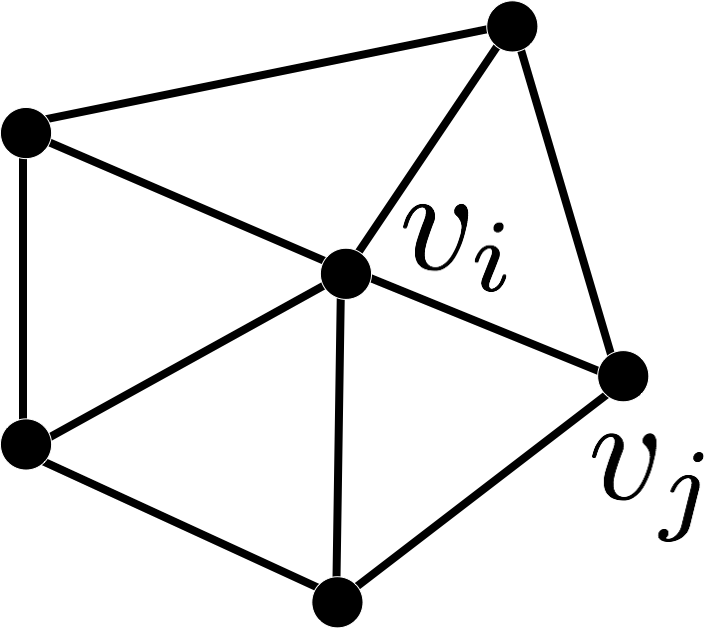# Cotan Laplace Discretization

$\laplace \vec{x}\of{v_i} \;:=\; \frac{1}{2A\of{v_i}} \sum_{v_j \in \set{N}_1\of{v_i}} \left( \cot \alpha_{ij} + \cot \beta_{ij} \right) \left( \vec{x}\of{v_j} - \vec{x}\of{v_i} \right)$

• Properties
• takes geometry and connectivity into account
• more accurate discretization
• can be derived through FEM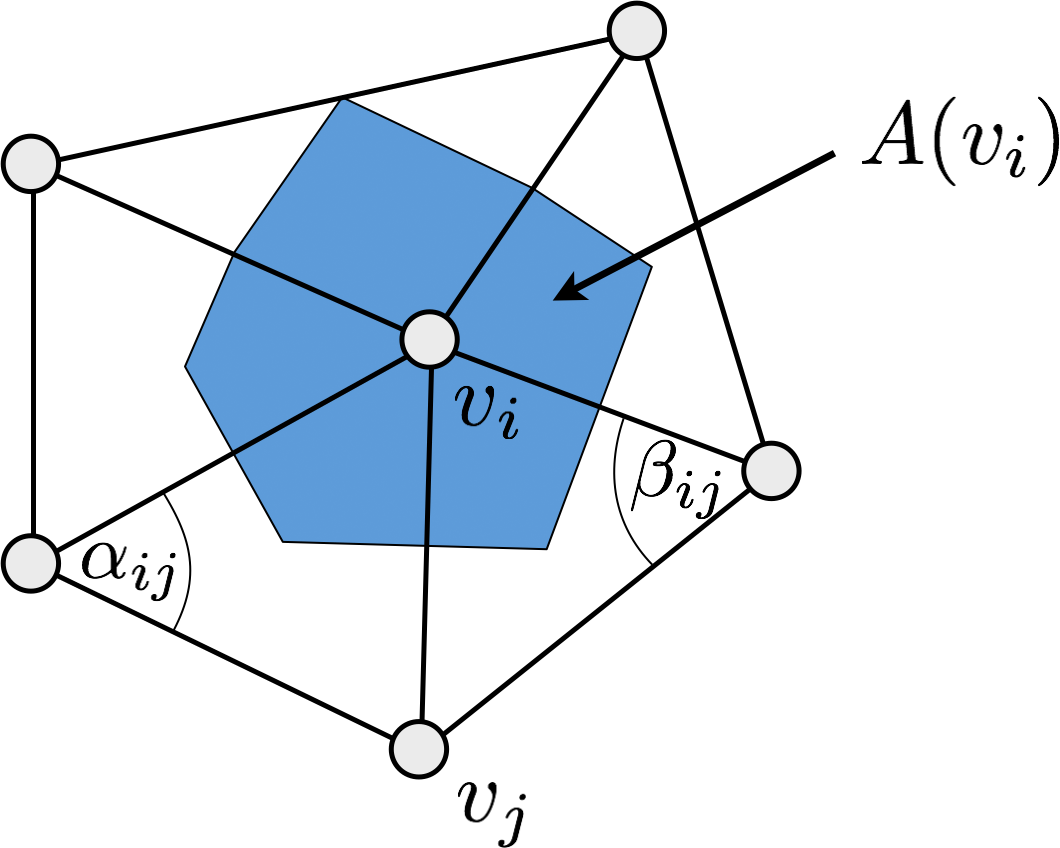# Uniform or Cotan Discretization?

• Uniform Laplacian is an inaccurate discretization
• Might be non-zero even for planar meshes
• Smoothes geometry and triangulation
• Might be desired for mesh regularization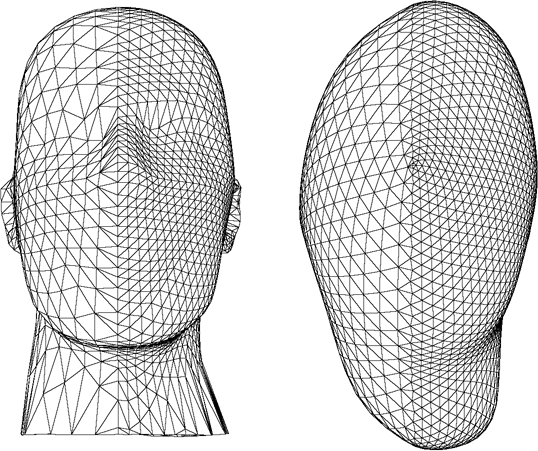# Numerical Integration

• Let’s write the position update in matrix notation
• Write all points $$\vec{x}_i^{(t)}$$ in a large vector/matrix: $\vec{X}^{(t)} = \trans{\left( \vec{x}_1^{(t)}, \ldots, \vec{x}_n^{(t)} \right)} \in \R^{n\times 3}$
• Matrix version of explicit integration $\vec{X}^{(t+1)} = (\vec{I} + \delta t \, \lambda \vec{L}) \, \vec{X}^{(t)}$
• Matrix version of implicit integration $(\vec{I} - \delta t \, \lambda \vec{L}) \, \vec{X}^{(t+1)} = \vec{X}^{(t)}$
Easy to implement, but requires small $$\delta t \lambda$$ for stability
Works for any $$\delta t \lambda$$, but has to solve linear system(s)

# Isotropic Triangle Remeshing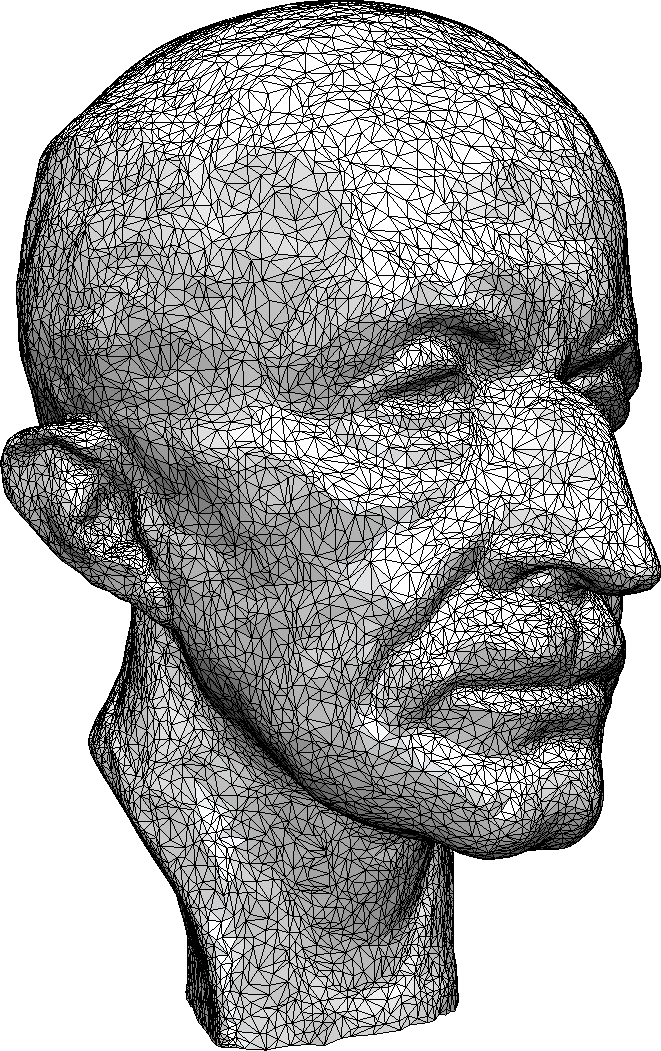original mesh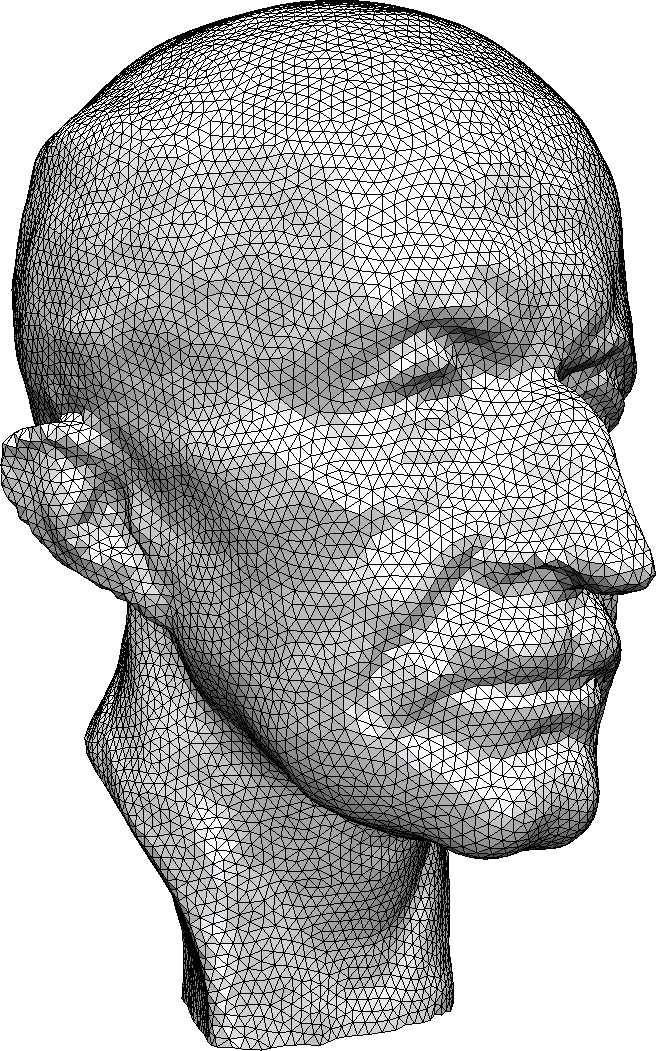uniform remeshing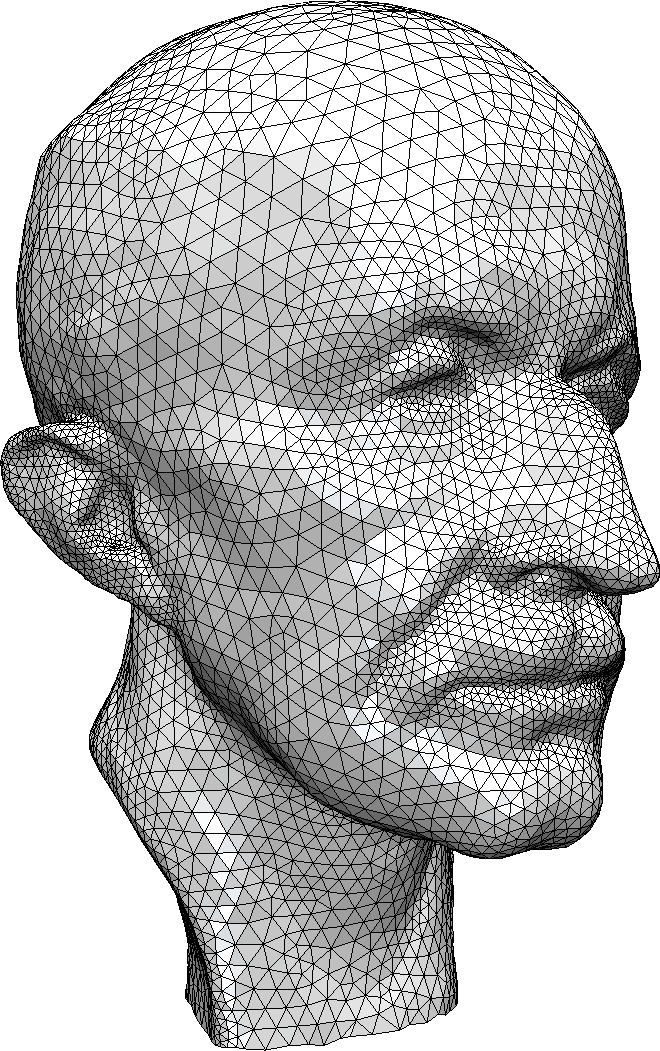adaptive remeshing

# Uniform Remeshing

• Specify target edge length $$L$$
• Iterate a few times
1. Split edges longer than $$\frac{4}{3} L$$
2. Collapse edges shorter than $$\frac{4}{5}L$$
3. Flip edges to get closer to valence 6
4. Shift vertices by tangential relaxation
5. Project vertices onto input mesh

# Local Remeshing Operators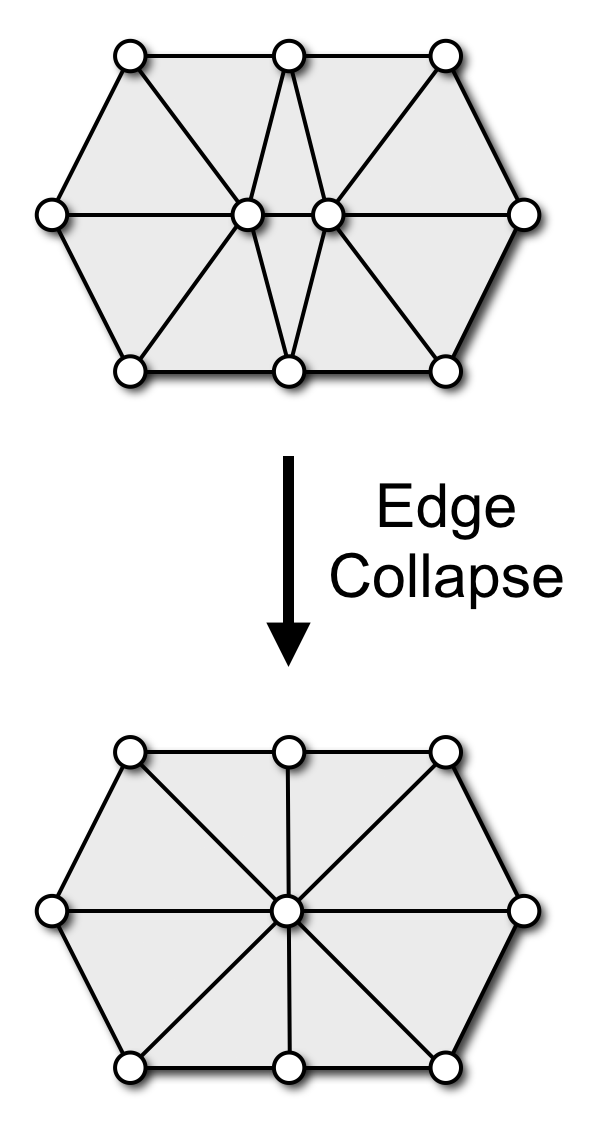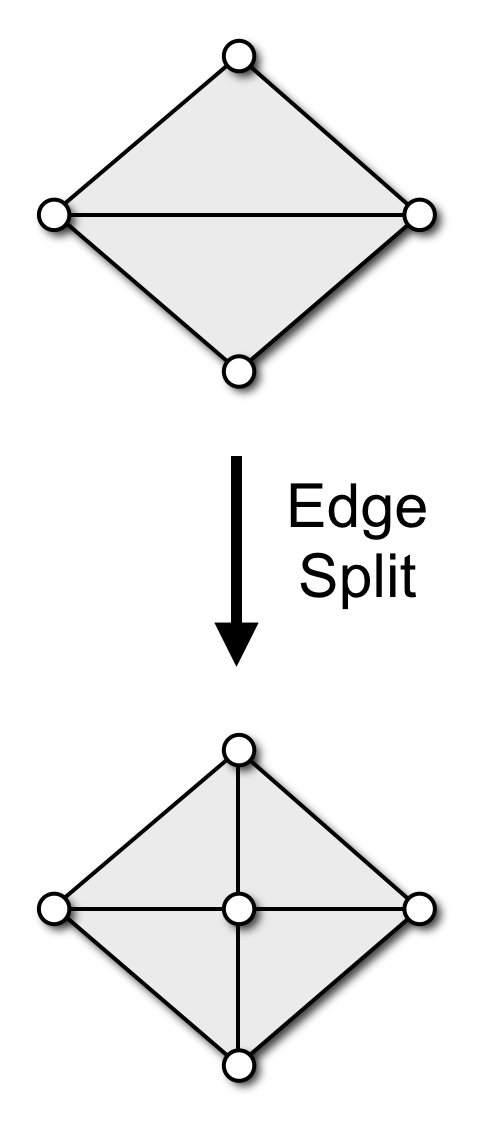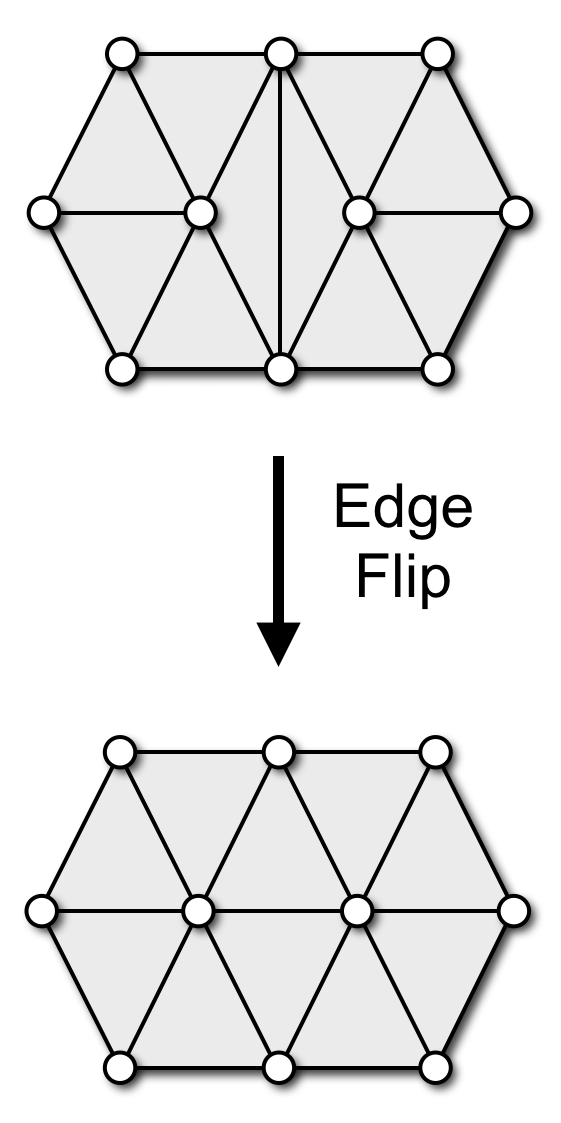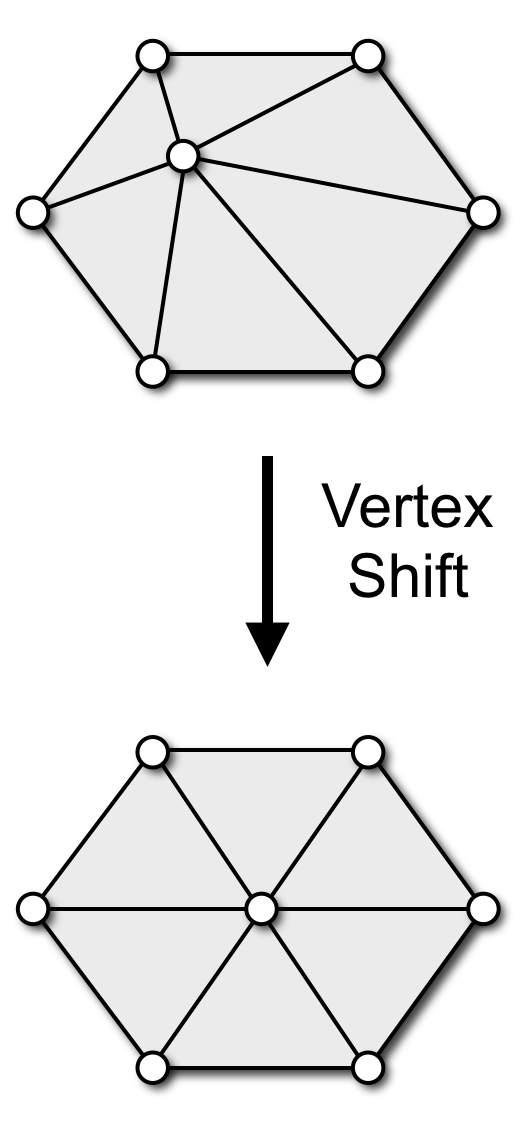# Adaptive Remeshing

• Adapt edge length to local curvature
• Compute maximum principle curvature on reference mesh
• Determine local target edge length from max-curvature
• Adjust split & collapse criteria accordingly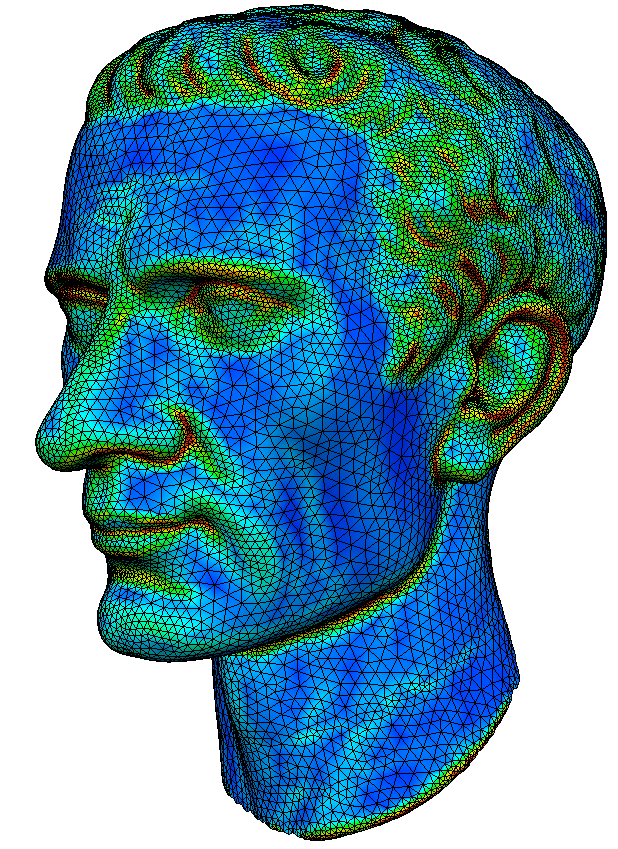# Feature Preservation

• Define feature edges / vertices
• Large dihedral angles
• Material boundaries
• Adjust local operators
• Don’t flip feature edges
• Collapse only along features
• Univariate smoothing
• Project to feature curves
• Don’t touch feature vertices